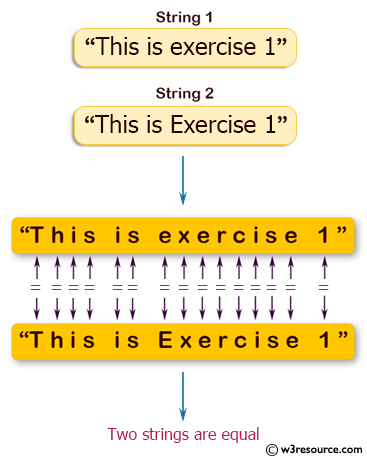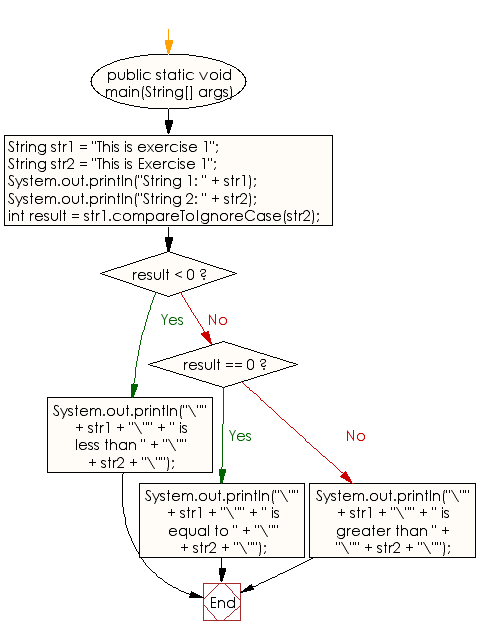﻿ Java - Compare two strings lexicographically, ignoring case

# Java: Compare two strings lexicographically, ignoring case differences

## Java String: Exercise-6 with Solution

Write a Java program to compare two strings lexicographically, ignoring case differences.

Visual Presentation:Sample Solution:

Java Code:

``````// Define a public class named Exercise6.
public class Exercise6 {
// Define the main method.
public static void main(String[] args) {
// Declare and initialize two string variables, str1 and str2.
String str1 = "This is exercise 1";
String str2 = "This is Exercise 1";

// Print the first string.
System.out.println("String 1: " + str1);
// Print the second string.
System.out.println("String 2: " + str2);

// Compare the two strings ignoring case sensitivity and get the result of the comparison.
int result = str1.compareToIgnoreCase(str2);

// Display the results of the comparison.
if (result < 0) {
// If str1 (ignoring case) is less than str2 (ignoring case).
System.out.println("\"" + str1 + "\"" +
" is less than " +
"\"" + str2 + "\"");
} else if (result == 0) {
// If str1 (ignoring case) is equal to str2 (ignoring case).
System.out.println("\"" + str1 + "\"" +
" is equal to " +
"\"" + str2 + "\"");
} else { // if (result > 0)
// If str1 (ignoring case) is greater than str2 (ignoring case).
System.out.println("\"" + str1 + "\"" +
" is greater than " +
"\"" + str2 + "\"");
}
}
}
```
```

Sample Output:

```String 1: This is exercise 1
String 2: This is Exercise 1
"This is exercise 1" is equal to "This is Exercise 1"
```

Flowchart:Java Code Editor:

Improve this sample solution and post your code through Disqus

What is the difficulty level of this exercise?

Test your Programming skills with w3resource's quiz.

﻿